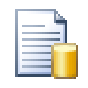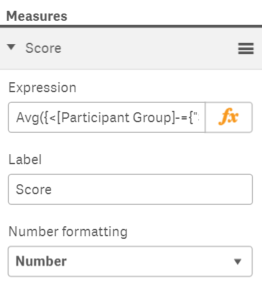# QlikView App Dev

Discussion Board for collaboration related to QlikView App Development.

cancel
Showing results for
Did you mean:Partner - Specialist

## Shorter "Background Color" Expressions?

I have a bar chart with one dimension and measure and I would like to color each bar according to its expression value. The expression is:

AVG({<[Question Response Group ID]={"5"}>} [Answer Choice Value])

When I go to style the Background Color, I have this obnoxious expression:

IF(AVG({<[Question Response Group ID]={"5"}>} [Answer Choice Value])=0,\$(vYellow),

IF(AVG({<[Question Response Group ID]={"5"}>} [Answer Choice Value])>0,

IF(AVG({<[Question Response Group ID]={"5"}>} [Answer Choice Value])<=2,

IF(AVG({<[Question Response Group ID]={"5"}>} [Answer Choice Value])>1,\$(vLightGreen),\$(vGreenYellow)),\$(vGreen)),

IF(AVG({<[Question Response Group ID]={"5"}>} [Answer Choice Value])>=-2,

IF(AVG({<[Question Response Group ID]={"5"}>} [Answer Choice Value])<-1,\$(vOrange),\$(vOrangeYellow)),\$(vRed))))

Is there a way I can build this definition without repeating the expression 6 times? Am I missing some EXPRESSIONVALUE function or something that would make this considerably more manageable (I have other definitions that are much worse)?

18 RepliesMVP

It should work the same in Qlik Sense. Have you encountered any issues?

-Rob

http://qlikviewcookbook.comPartner - Specialist
Author

I am not able to use the measure label as a column in Qlik Sense 3.0 as in QlikView. Perhaps there is a different syntax?MVP

Tim,

I've not encountered any problem referencing column labels in Qlik Sense desktop 3.0.  Can you provide an example or more detail on the problem you are seeing?

-Rob

http://qlikviewcookbook.comPartner - Specialist
Author

I'm on Qlik Sense Enterprise 3.0. I have a bar chart with one dimension and a Measure with the Label "Score" and the expression below.Avg({<[Participant Group]-={"Self"}>}[Answer Choice Value])

Then, I have a huge expression for the Colors and Legends attribute that duplicates that formula many times. I am not able to substitute the field [Score] in for the formula like I can in QlikView. Is there another syntax to do this?MVP

Perhaps the issue is in your color expression. Note that you can't use "Score" as a function argument. Can you pos the color expression?

-RobPartner - Specialist
Author

IF(Avg({<[Participant Group]-={"Self"}>}[Answer Choice Value]),vYellow,

IF(Avg({<[Participant Group]-={"Self"}>}[Answer Choice Value])>0,

IF(Avg({<[Participant Group]-={"Self"}>}[Answer Choice Value])<=2,

IF(Avg({<[Participant Group]-={"Self"}>}[Answer Choice Value])>1,vLightGreen,vGreenYellow),vScoreGreen),

IF(Avg({<[Participant Group]-={"Self"}>}[Answer Choice Value])>=-2,

IF(Avg({<[Participant Group]-={"Self"}>}[Answer Choice Value])<-1,vOrange,vOrangeYellow),vScoreRed)))MVP

Can you post the color expression you are using when you use the "Score" label? (I take it the one you posted is the one that works).

-Rob

http://qlikviewcookbook.comPartner - Specialist
Author

That's the thing that doesn't work in Qlik Sense which works in QlikView. In QlikView I can substitute [Score] instead of Avg({<[Participant Group]-={"Self"}>}[Answer Choice Value]). Here I tried the exact same thing, but it does not work in Qlik Sense. I am stuck with the long version.Partner - Specialist
Author

Finally arrived at a much simpler solution for Qlik Sense. I am using the PICK and CEIL functions to build this expression:

PICK(CEIL(AVG({<[Question Response Group ID]={"5"}>} [Answer Choice Value])+4),

vRed,

vOrange,

vOrangeYellow,

vYellow,

vGreenYellow,

vLightGreen,

vScoreGreen

)

Which is notably more efficient in space and resource usage.